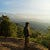# Does text similarity metrics help in text classification problems?

There was one news spreading all over the internet, “Sanitizers Don’t Help Against Coronavirus”. This news was shared on social media with a reasoning that because coronavirus is a virus and anti-bacterial hand sanitizer won’t do anything. According to FactCheck.org, the rumor started on Twitter when a self-identified “scientist” posted their theory on March 1, 2020. However, according to the CDC, using a hand sanitizer that contains at least 60 percent alcohol is a suitable substitute if there is no soap and water available.

There was another story going around, “The federal government has mandated that workplaces close for two…

# Building an Artificial Neural Network using Tensorflow 2.0

In this notebook, we will see how to implement an Artificial Neural Network using Tensorflow 2.0. We will be using Fashion MNIST dataset directly importing it from Tensorflow datasets

# Importing libraries

`import numpy as npimport datetimeimport tensorflow as tffrom tensorflow.keras.datasets import fashion_mnisttf.__version__'2.3.0'`

# Data preprocessing

`(X_train, y_train),(X_test, y_test)=fashion_mnist.load_data()Downloading data from https://storage.googleapis.com/tensorflow/tf-keras-datasets/train-labels-idx1-ubyte.gz 32768/29515 [=================================] - 0s 3us/step Downloading data from https://storage.googleapis.com/tensorflow/tf-keras-datasets/train-images-idx3-ubyte.gz 26427392/26421880 [==============================] - 12s 0us/step Downloading data from https://storage.googleapis.com/tensorflow/tf-keras-datasets/t10k-labels-idx1-ubyte.gz 8192/5148 [===============================================] - 0s 0us/step Downloading data from https://storage.googleapis.com/tensorflow/tf-keras-datasets/t10k-images-idx3-ubyte.gz 4423680/4422102 [==============================] - 2s 0us/step…`

# Tensorflow 2.0 basics

In this notebook we see the basics of Tensorflow 2.0. The latest tensorflow version during the time of this notebook prep was 2.4.0. To install tensorflow or tensorflow-gpu on your system, please follow this link

`import tensorflow as tftf.__version__'2.4.0'`

# Constant

We define a simple constant using tf.constant() function

`# Defning a constanttensor=tf.constant([[2,4], [5,6]])tensor<tf.Tensor: shape=(2, 2), dtype=int32, numpy=array([[2, 4],       [5, 6]], dtype=int32)>`

We can also retrieve the value from tensor constant. The idea is to extract the value using numpy() function

`# Getting value of constanttensor.numpy()array([[2, 4],       [5, 6]], dtype=int32)`

We can also convert…

# Linear Regression

Linear regression attempts to model the connection between two variables by fitting a linear equation to the observed data. One variable is taken into account as an explanatory variable, while the opposite is taken into account to be a dependent variable. Let us consider two variables X and Y and that we want to relate the Y to X employing a rectilinear regression model.

`x=[,,,[3.5]]y=[,,,[7.5]]`

Before even trying any linear model, one should first find out if there is a relationship between the variables. One can use the scatter plot in determining such a relationship. …## Abhishek Koirala

Lead NLP Product Engineer at Diyo.ai Pvt. Ltd. Interested in machine learning research